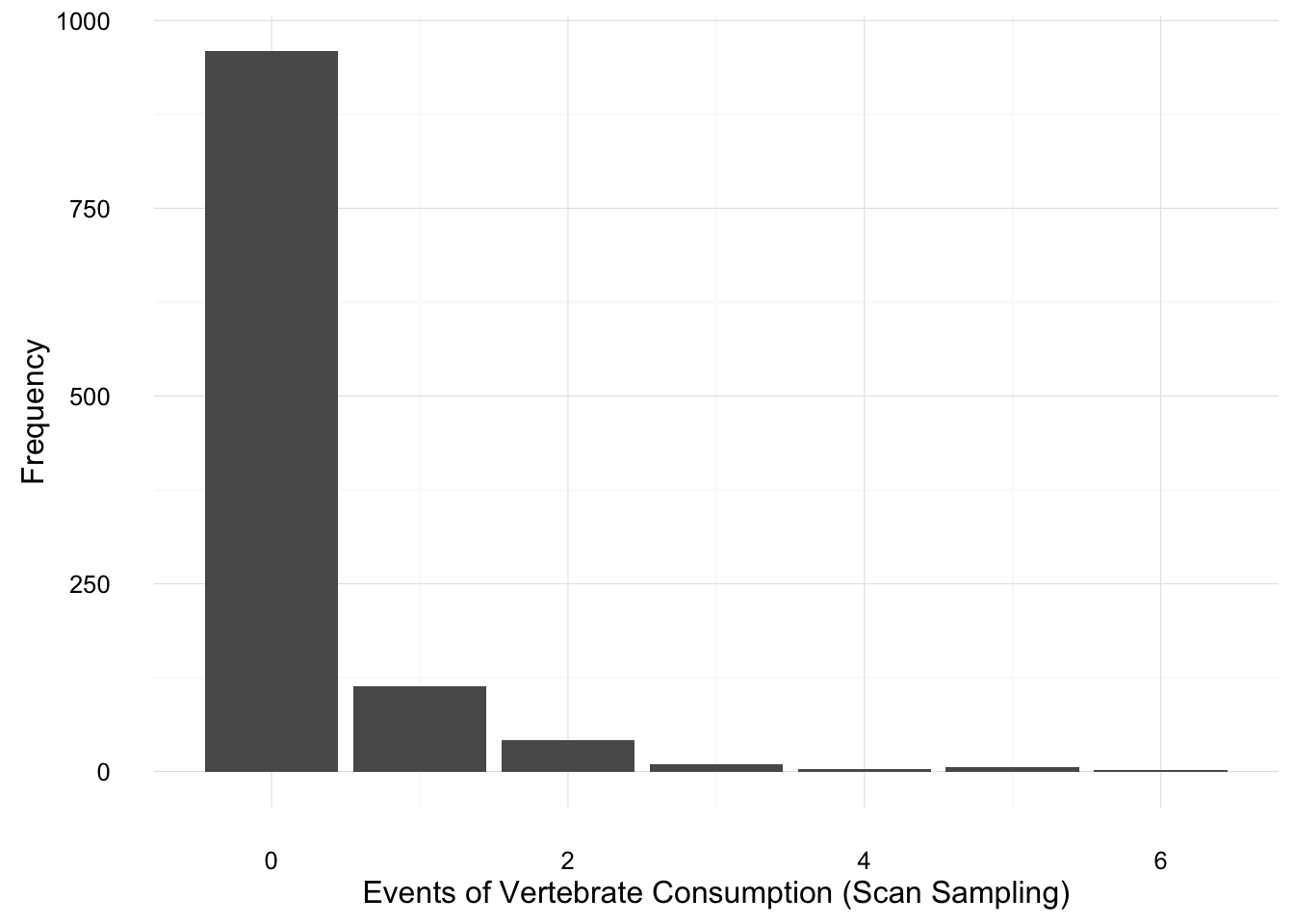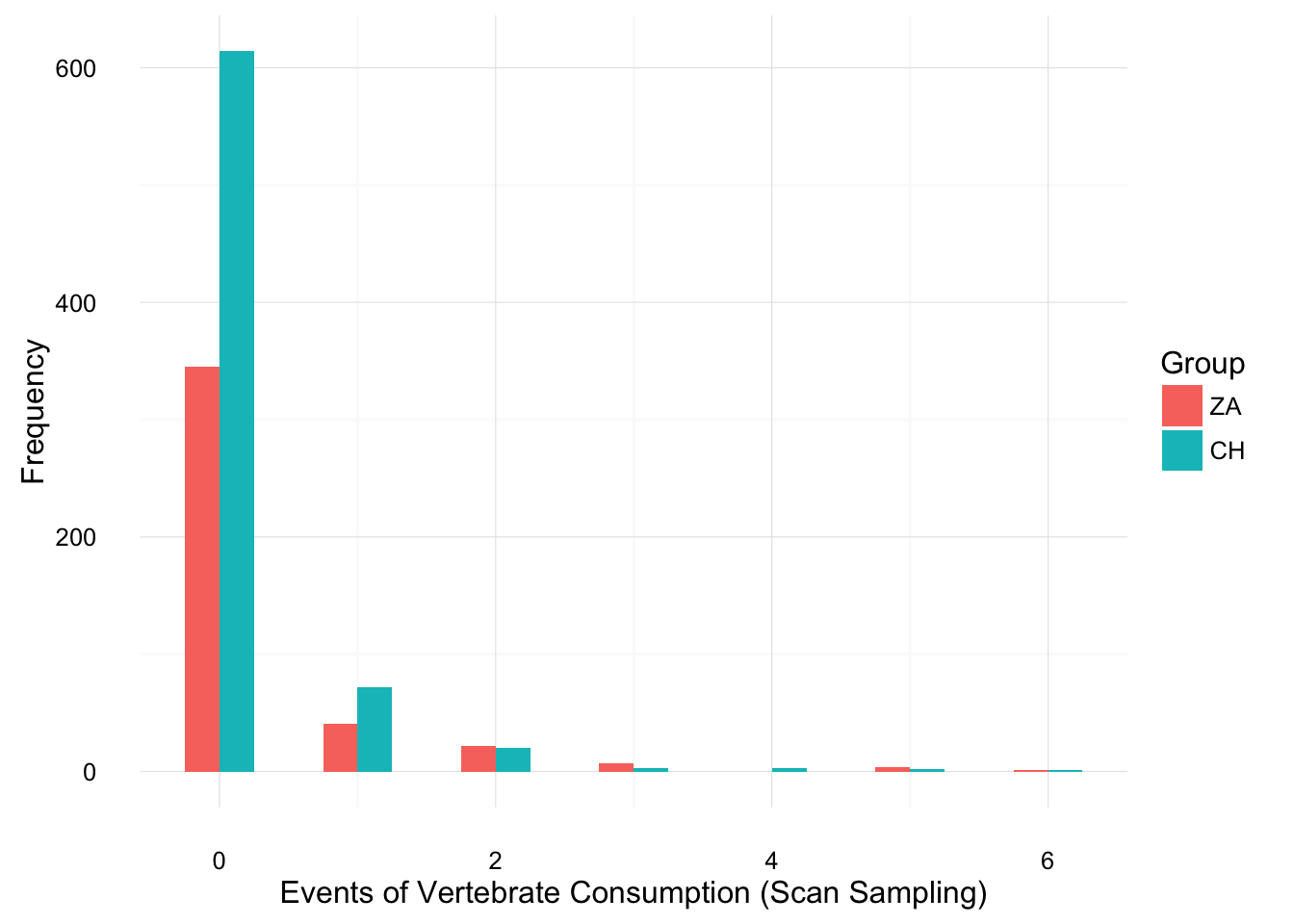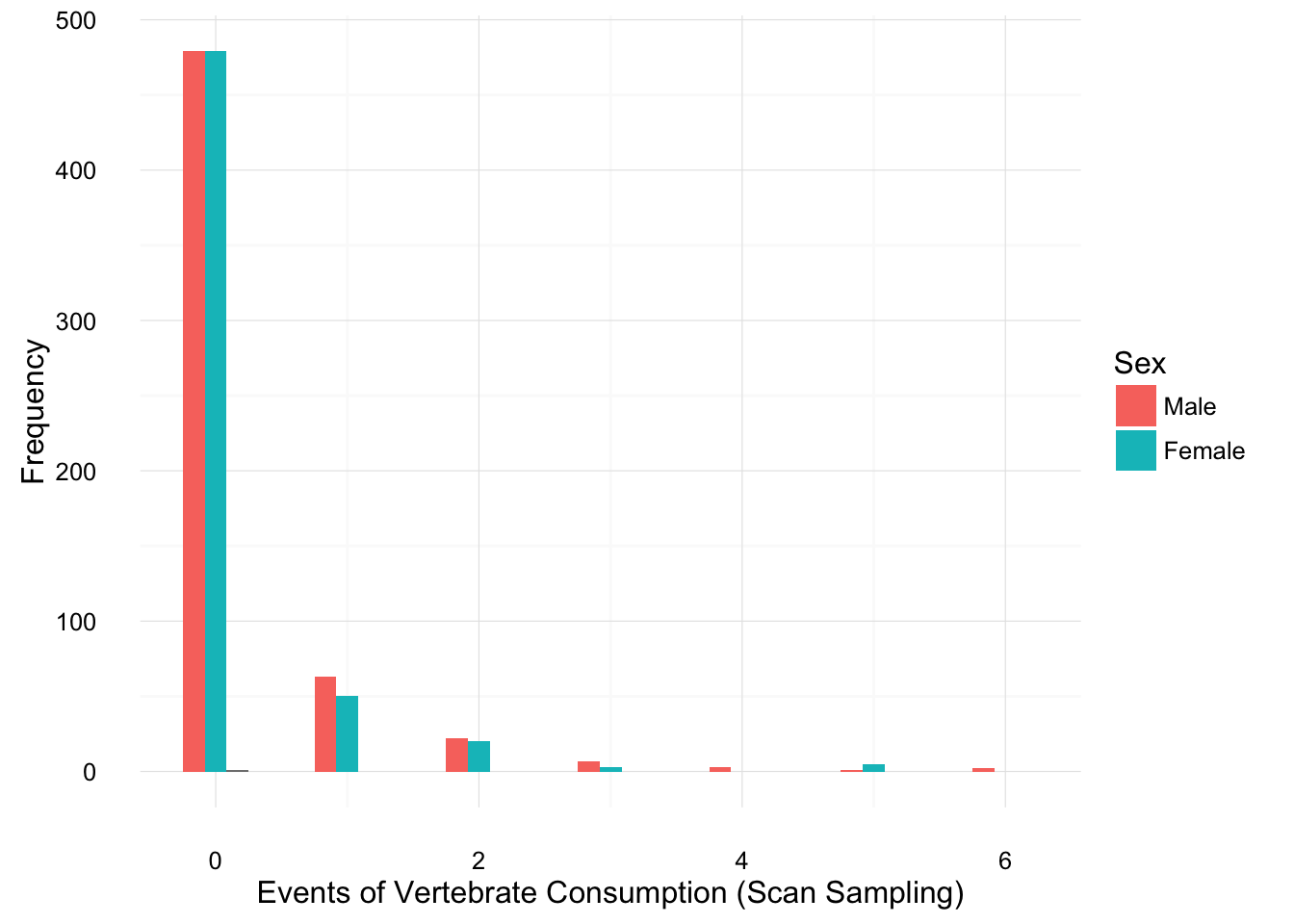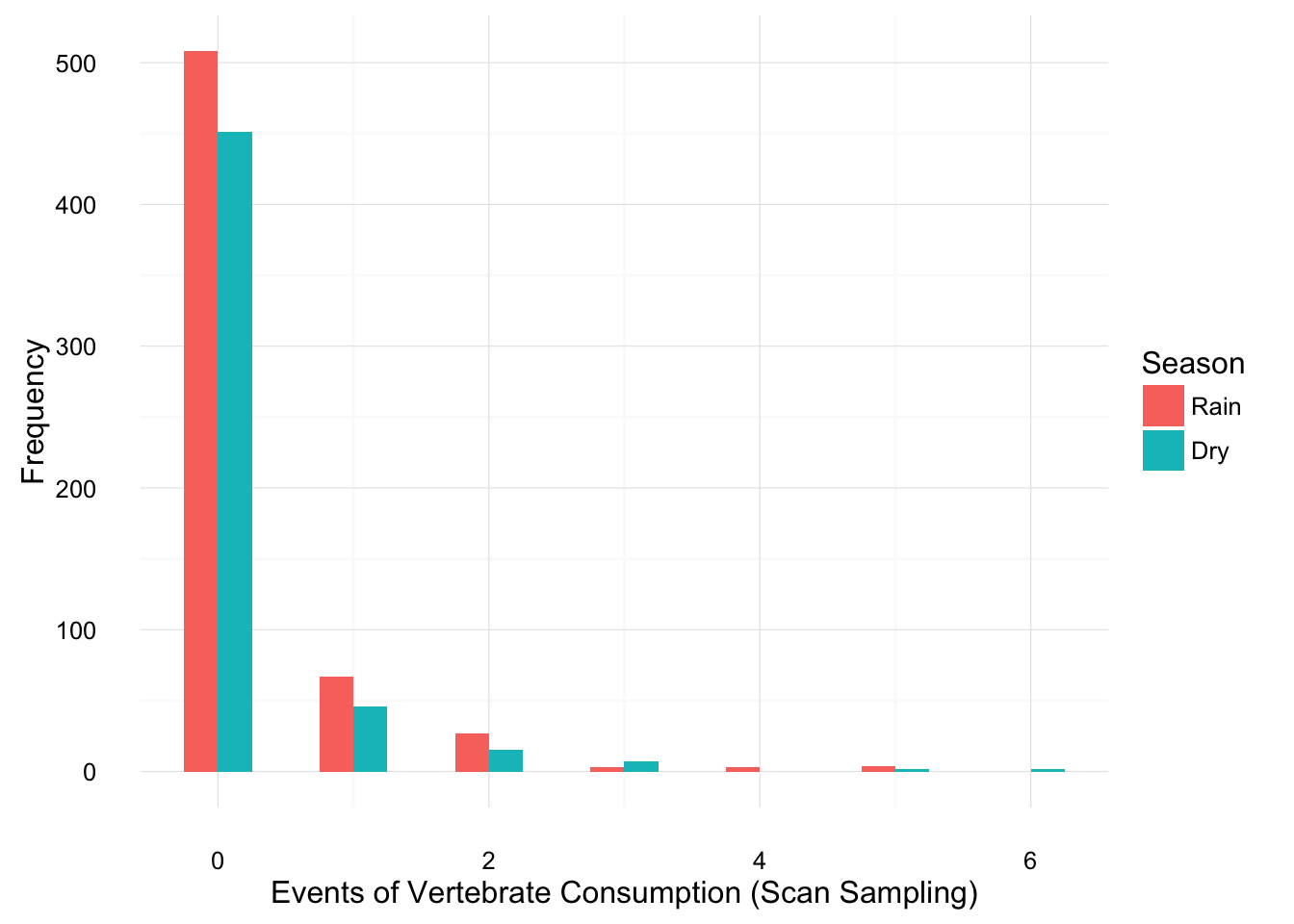## Vertebrate consumption by wild bearded capuchin monkeys (Sapajus libidinosus) living in a Brazilian semiarid habitat

#### Short report from a data analysis in R

Let’s pull all data

``````SSdata <- read.csv2("~/Desktop/CVP/data2SS.csv") # Reading dataset with Scan Sampling raw data

## Data cleaning

Some data cleaning in order to improve readability

``````SSdata\$Group <- as.factor(SSdata\$Group) ; AOdata\$Group <- as.factor(AOdata\$Group)
levels(SSdata\$Group) <- c('ZA', 'CH'); levels(AOdata\$Group) <- c('ZA', 'CH');
SSdata\$Sex <- as.factor(SSdata\$Sex)
levels(SSdata\$Sex) <- c('Male', 'Female')
SSdata\$Age <- as.factor(SSdata\$Age)
SSdata\$Season <- as.factor(SSdata\$Season)
levels(SSdata\$Season) <- c('Rain', 'Dry')``````

## Exercises

Correct and fill: A total of 474 events of vertebrate consumption were observed between 2006 and 2010 (274 recorded by scan sampling and 200 recorded by all occurrences), CH group accounted for XX events, and ZA group for XX events.

``sum(SSdata\$Scan.consumed.month) + sum(AOdata\$TotalEpisodes)``
``##  446``
``sum(SSdata\$Scan.consumed.month)``
``##  281``
``sum(AOdata\$TotalEpisodes)``
``##  165``
``sum(AOdata\$TotalEpisodes[AOdata\$Group == 'CH']) + sum(SSdata\$Scan.consumed.month[SSdata\$Group == 'CH'])``
``##  212``
``sum(AOdata\$TotalEpisodes[AOdata\$Group == 'ZA']) + sum(SSdata\$Scan.consumed.month[SSdata\$Group == 'ZA'])``
``##  234``

A total of 446 events of vertebrate consumption were observed between 2006 and 2010 (281 recorded by scan sampling and 165 recorded by all occurrences), CH group accounted for 212 events, and ZA group for 234 events.

Correct and fill: Of 213 episodes recorded by all occurrences for both groups, 81.2% (N=XX) were episodes in which only one individual was seen consuming the prey, whereas XX% (N=XX) were episodes in which two or more monkeys were seen consuming the same prey;

``sum(AOdata\$IndEpisodes)/sum(AOdata\$TotalEpisodes)*100 # percent``
``##  81.21212``
``sum(AOdata\$IndEpisodes) # n``
``##  134``
``sum(AOdata\$MultipleIndEpisodes)/sum(AOdata\$TotalEpisodes)*100 # percent``
``##  18.78788``
``sum(AOdata\$MultipleIndEpisodes) # n``
``##  31``

Of 165 episodes recorded by all occurrences for both groups, 81.21% (N=134) were episodes in which only one individual was seen consuming the prey, whereas 18.79% (N=31) were episodes in which two or more monkeys were seen consuming the same prey;

Correct and fill: The major prey types were reptiles (n=131: lizards, geckos, snakes, iguanas), mammals (n=95: primarily rodents, but also opossums, a bat) and birds (n=34: especially nestlings and eggs).

``sum(c(SSdata\$Lizards, SSdata\$Serpents)) # reptiles``
``##  136``
``sum(c(SSdata\$Rodents, SSdata\$MarsupialsMammals, SSdata\$Chiroptera)) # mammals``
``##  97``
``sum(SSdata\$Aves) # birds``
``##  34``

The major prey types were reptiles (n=136: lizards, geckos, snakes, iguanas), mammals (n=97: primarily rodents, but also opossums, a bat) and birds (n=34: especially nestlings and eggs).

Calculate percentages for Table 2

Table 2 in the Word file as many errors. Here I recalculate all values for paper-corrections.

``str(SSdata[,9:15])``
``````## 'data.frame':    1135 obs. of  7 variables:
##  \$ Lizards          : int  0 0 0 1 4 0 0 0 0 2 ...
##  \$ Serpents         : int  0 0 0 0 0 0 0 0 0 0 ...
##  \$ Rodents          : int  0 0 0 0 0 0 0 0 0 0 ...
##  \$ MarsupialsMammals: int  0 0 0 0 0 0 0 0 0 0 ...
##  \$ Chiroptera       : int  0 0 0 0 0 0 0 0 0 0 ...
##  \$ Aves             : int  0 0 0 0 0 0 0 0 0 0 ...
##  \$ Undertermined    : int  0 0 0 0 1 0 0 0 0 0 ...``````
``sum(SSdata\$Lizards)/sum(SSdata[,9:15])*100``
``##  43.77224``
``sum(SSdata\$Serpents)/sum(SSdata[,9:15])*100``
``##  4.626335``
``sum(SSdata\$Rodents)/sum(SSdata[,9:15])*100``
``##  28.46975``
``sum(SSdata\$MarsupialsMammals)/sum(SSdata[,9:15])*100``
``##  5.338078``
``sum(SSdata\$Chiroptera)/sum(SSdata[,9:15])*100``
``##  0.7117438``
``sum(SSdata\$Aves)/sum(SSdata[,9:15])*100``
``##  12.09964``
``sum(SSdata\$Undertermined)/sum(SSdata[,9:15])*100``
``##  4.982206``
``sum(SSdata[,9:15])``
``##  281``

## Statistical modelling

Our dependent variable (vertebrate consumption events) is a count type of variable (goes from integer 0, to 6):

``````library(ggplot2)
ggplot(SSdata, aes(Scan.consumed.month)) +
geom_bar() +
labs(x = 'Events of Vertebrate Consumption (Scan Sampling)', y = 'Frequency') +
theme_minimal()``````The distribution of counts is discrete, not continuous, and is limited to non-negative values, a very popular case of this is the Poisson distribution. And here it could be modeled as follows:

``````fit.p <- glm(Scan.consumed.month ~ Group + Season + Sex + Age, data = SSdata, family = poisson)

summary(fit.p) # Poisson Regression``````
``````##
## Call:
## glm(formula = Scan.consumed.month ~ Group + Season + Sex + Age,
##     family = poisson, data = SSdata)
##
## Deviance Residuals:
##     Min       1Q   Median       3Q      Max
## -0.9565  -0.7480  -0.6629  -0.5617   5.2424
##
## Coefficients:
##             Estimate Std. Error z value Pr(>|z|)
## (Intercept)  -0.7821     0.1452  -5.386 7.20e-08 ***
## GroupCH      -0.4918     0.1226  -4.010 6.06e-05 ***
## SeasonDry    -0.1859     0.1210  -1.536 0.124523
## SexFemale    -0.3314     0.1242  -2.668 0.007625 **
## AgeJuvenile  -0.0556     0.1242  -0.447 0.654528
## AgeInfant    -1.3681     0.3884  -3.522 0.000428 ***
## ---
## Signif. codes:  0 '***' 0.001 '**' 0.01 '*' 0.05 '.' 0.1 ' ' 1
##
## (Dispersion parameter for poisson family taken to be 1)
##
##     Null deviance: 1139.3  on 1133  degrees of freedom
## Residual deviance: 1100.9  on 1128  degrees of freedom
##   (1 observation deleted due to missingness)
## AIC: 1516.6
##
## Number of Fisher Scoring iterations: 6``````
``anova(fit.p) # ANOVA of the Poisson model``
``````## Analysis of Deviance Table
##
##
## Response: Scan.consumed.month
##
## Terms added sequentially (first to last)
##
##
##        Df Deviance Resid. Df Resid. Dev
## NULL                    1133     1139.3
## Group   1  11.7729      1132     1127.5
## Season  1   2.1014      1131     1125.4
## Sex     1   5.0804      1130     1120.3
## Age     2  19.4230      1128     1100.9``````

However, a Poisson distribution assumes that mean = variance.

``var(SSdata\$Scan.consumed.month)``
``##  0.5039073``
``mean(SSdata\$Scan.consumed.month)``
``##  0.2475771``

Which is clearly not our case. This causes a particular problem typical of count data modelling (i.e. overdispersion), and usually leads to a downwards bias on the estimation of standard errors. A more general form of the Poisson distribution, that allows for non-equal variance and mean is the Negative Binomial Distribution.

``````fit.nb <- glm.nb(Scan.consumed.month ~ Group + Season + Sex + Age, data = SSdata) # This function has an extra parameter Theta, which corrects for bias caused by Poisson Regression assumption.

summary(fit.nb) # Negative Binomial Regression``````
``````##
## Call:
## glm.nb(formula = Scan.consumed.month ~ Group + Season + Sex +
##     Age, data = SSdata, init.theta = 0.2609052582, link = log)
##
## Deviance Residuals:
##     Min       1Q   Median       3Q      Max
## -0.7485  -0.6230  -0.5571  -0.4782   2.7305
##
## Coefficients:
##             Estimate Std. Error z value Pr(>|z|)
## (Intercept)  -0.6883     0.2155  -3.194  0.00140 **
## GroupCH      -0.5562     0.1765  -3.152  0.00162 **
## SeasonDry    -0.2404     0.1717  -1.400  0.16143
## SexFemale    -0.3905     0.1765  -2.213  0.02691 *
## AgeJuvenile  -0.0662     0.1781  -0.372  0.71012
## AgeInfant    -1.3778     0.4492  -3.067  0.00216 **
## ---
## Signif. codes:  0 '***' 0.001 '**' 0.01 '*' 0.05 '.' 0.1 ' ' 1
##
## (Dispersion parameter for Negative Binomial(0.2609) family taken to be 1)
##
##     Null deviance: 545.25  on 1133  degrees of freedom
## Residual deviance: 522.90  on 1128  degrees of freedom
##   (1 observation deleted due to missingness)
## AIC: 1341.5
##
## Number of Fisher Scoring iterations: 1
##
##
##               Theta:  0.2609
##           Std. Err.:  0.0411
##
##  2 x log-likelihood:  -1327.5090``````
``anova(fit.nb) # ANOVA of Negative Binomial model``
``## Warning in anova.negbin(fit.nb): tests made without re-estimating 'theta'``
``````## Analysis of Deviance Table
##
## Model: Negative Binomial(0.2609), link: log
##
## Response: Scan.consumed.month
##
## Terms added sequentially (first to last)
##
##
##        Df Deviance Resid. Df Resid. Dev Pr(>Chi)
## NULL                    1133     545.25
## Group   1   5.9619      1132     539.28 0.014618 *
## Season  1   1.7121      1131     537.57 0.190715
## Sex     1   3.4963      1130     534.07 0.061505 .
## Age     2  11.1759      1128     522.90 0.003743 **
## ---
## Signif. codes:  0 '***' 0.001 '**' 0.01 '*' 0.05 '.' 0.1 ' ' 1``````

#### Checking assumptions

Negative binomial models assume the conditional means are not equal to the conditional variances. This inequality is captured by estimating a dispersion parameter (not shown in the output) that is held constant in a Poisson model. Thus, the Poisson model is actually nested in the negative binomial model. We can then use a likelihood ratio test to compare these two and test this model assumption.

``````Comparison <- 2 * (logLik(fit.nb) - logLik(fit.p)) # 2 times the difference in log likelihoods
Comparison # likelihood ratio test to compare these two and test this model assumption``````
``## 'log Lik.' 177.0835 (df=7)``
``````ch.test <- pchisq(Comparison, df = 1, lower.tail = FALSE) # in the case of nested models is distributed as chi-square with degrees of freedom equal to the difference in the degrees of freedom of the two models, here df=7-6=1.
ch.test # This very large chi-square strongly suggests the negative binomial model, which estimates the dispersion parameter, is more appropriate than the Poisson model.``````
``## 'log Lik.' 2.100128e-40 (df=7)``
``est <- cbind(Estimate = coef(fit.nb), confint(fit.nb)) # We can get the confidence intervals for the coefficients by profiling the likelihood function.``
``## Waiting for profiling to be done...``
``est``
``````##               Estimate      2.5 %      97.5 %
## (Intercept) -0.6882875 -1.1371506 -0.23139095
## GroupCH     -0.5562141 -0.9152036 -0.20159162
## SeasonDry   -0.2404277 -0.5855178  0.10245693
## SexFemale   -0.3904951 -0.7434851 -0.04125851
## AgeJuvenile -0.0662041 -0.4161899  0.28247931
## AgeInfant   -1.3777459 -2.3355207 -0.54251151``````
``exp(est) # We might be interested in looking at incident rate ratios rather than coefficients. To do this, we can exponentiate our model coefficients. The same applies to the confidence intervals.``
``````##              Estimate      2.5 %    97.5 %
## (Intercept) 0.5024357 0.32073161 0.7934292
## GroupCH     0.5733757 0.40043508 0.8174287
## SeasonDry   0.7862915 0.55681746 1.1078896
## SexFemale   0.6767218 0.47545401 0.9595810
## AgeJuvenile 0.9359398 0.65955499 1.3264143
## AgeInfant   0.2521463 0.09676009 0.5812865``````

## Chi-square tests

#### Differences of vertebrate consumption (from SS data) amongst groups

``````ggplot(SSdata, aes(Scan.consumed.month, fill = Group)) +
geom_histogram(binwidth=.5, position="dodge") +
labs(x = 'Events of Vertebrate Consumption (Scan Sampling)', y = 'Frequency') +
theme_minimal()````````````# Create a contigency table

M <- matrix(c(sum(SSdata\$Scan.consumed.month[SSdata\$Group == 'CH'], na.rm = T), sum(SSdata\$Scan.consumed.month[SSdata\$Group == 'ZA'], na.rm = T)))
Xsq <- chisq.test(M)
Xsq\$observed   # observed counts (same as M)``````
``##  149 132``
``Xsq\$expected   # expected counts under the null``
``##  140.5 140.5``
``Xsq\$residuals  # Pearson residuals``
``##   0.7171017 -0.7171017``
``Xsq\$stdres     # standardized residuals``
``##   1.014135 -1.014135``
``Xsq``
``````##
##  Chi-squared test for given probabilities
##
## data:  M
## X-squared = 1.0285, df = 1, p-value = 0.3105``````

Not significant!

#### Differences of vertebrate consumption (from SS data) amongst Sex

``````ggplot(SSdata, aes(Scan.consumed.month, fill = Sex)) +
geom_histogram(binwidth=.5, position="dodge") +
labs(x = 'Events of Vertebrate Consumption (Scan Sampling)', y = 'Frequency') +
theme_minimal()````````````# Create a contigency table

M <- matrix(c(sum(SSdata\$Scan.consumed.month[SSdata\$Sex == 'Male'], na.rm = T), sum(SSdata\$Scan.consumed.month[SSdata\$Sex == 'Female'], na.rm = T)))
Xsq <- chisq.test(M)
Xsq\$observed   # observed counts (same as M)``````
``##  157 124``
``Xsq\$expected   # expected counts under the null``
``##  140.5 140.5``
``Xsq\$residuals  # Pearson residuals``
``##   1.392021 -1.392021``
``Xsq\$stdres     # standardized residuals``
``##   1.968615 -1.968615``
``Xsq``
``````##
##  Chi-squared test for given probabilities
##
## data:  M
## X-squared = 3.8754, df = 1, p-value = 0.049``````

Significant!

#### Differences of vertebrate consumption (from SS data) amongst Seasons

``````ggplot(SSdata, aes(Scan.consumed.month, fill = Season)) +
geom_histogram(binwidth=.5, position="dodge") +
labs(x = 'Events of Vertebrate Consumption (Scan Sampling)', y = 'Frequency') +
theme_minimal()````````````# Create a contigency table

M <- matrix(c(sum(SSdata\$Scan.consumed.month[SSdata\$Season == 'Dry'], na.rm = T), sum(SSdata\$Scan.consumed.month[SSdata\$Season == 'Rain'], na.rm = T)))
Xsq <- chisq.test(M)
Xsq\$observed   # observed counts (same as M)``````
``##  119 162``
``Xsq\$expected   # expected counts under the null``
``##  140.5 140.5``
``Xsq\$residuals  # Pearson residuals``
``##  -1.813846  1.813846``
``Xsq\$stdres     # standardized residuals``
``##  -2.565165  2.565165``
``Xsq``
``````##
##  Chi-squared test for given probabilities
##
## data:  M
## X-squared = 6.5801, df = 1, p-value = 0.01031``````

Significant!

#### Differences of vertebrate consumption (from SS data) amongst Age groups

``````ggplot(SSdata, aes(Scan.consumed.month, fill = Age)) +
geom_histogram(binwidth=.5, position="dodge") +
labs(x = 'Events of Vertebrate Consumption (Scan Sampling)', y = 'Frequency') +
theme_minimal()``````